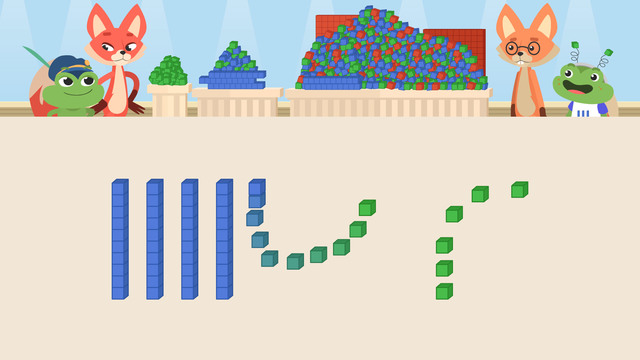# Regrouping Ones, Tens, and HundredsRating

Ø 5.0 / 1 ratings
The authorsTeam Digital
Regrouping Ones, Tens, and Hundreds
CCSS.MATH.CONTENT.3.NBT.A.2

## Basics on the topicRegrouping Ones, Tens, and Hundreds

Let's see how to regroup base ten blocks with Skylar and Henry!

### TranscriptRegrouping Ones, Tens, and Hundreds

Skylar and Henry are helping their friends get ready for the Blocks Rock exhibit they're working on for the Base Ten Appreciation week! In order to do that, they need to organize the base ten blocks. Let's help them by... Regrouping Ones, Tens, and Hundreds. When we have a large amount of base ten blocks, we can REGROUP them so they are easier to count. Since ten ones is the same as one ten, (...) we can regroup the ones to the tens place value. The process is called REgrouping because you are rearranging the blocks into different place value categories, or groups. There are ten tens in one hundred, (...) so we can regroup the tens to the hundreds place value. And there are ten hundreds in one thousand, (...) so we can regroup the hundreds to the thousands place value. Now, let's regroup the blocks for the first display. Here we have twenty-seven ones. How can we regroup the ones? (...) Regroup two TENS from twenty ones. How much do we have?(...) Two tens and seven ones is twenty-seven. What do you notice about the base ten blocks and twenty-seven? There is a two in the TENS place and there are two tens... and there is a seven in the ONES place and there are seven ones! Let's regroup the next display. When there are ones AND tens to regroup, start with the ones FIRST, so you can regroup all the tens at once. How do we regroup sixteen ones?(...) Regroup one TEN from sixteen ones. How much do we have now? (...) Now there are ten TENS and six ONES. How can we regroup the tens? (...) We can regroup ten TENS into one HUNDRED. How much do we have?(...) One hundred six. Let's help them with their last display. There are ten hundreds,(...) eighteen tens,(...) and thirty-eight ones.(...) How can we regroup the ones? (...) First, regroup thirty ones into three TENS. Now there are twenty-one TENS and eight ONES. How can we regroup the tens? (...) We can regroup twenty TENS into two HUNDREDS. Now there are twelve hundreds, one ten, and eight ones. How can we regroup the hundreds? (...) We can regroup ten HUNDREDS into one THOUSAND. How much do we have?(...) One thousand two hundred eighteen. Before we see how the displays turned out, let's summarize. Remember, (...) when we have a large amount of base ten blocks, we can regroup them so they are easier to count. Ten ones is the same as one ten... ten tens is the same as one hundred... and ten hundreds is the same as one thousand! "Phew! I'm ready to start celebrating!" "Yeah! I can't wait for the block party! And it looks like we finished EVERYTHING on time! Skylar and Henry had not finished everything. In fact, there were SO (...) MANY (...) MORE (....) BLOCKS.

## Regrouping Ones, Tens, and Hundreds exercise

Would you like to apply the knowledge you’ve learned? You can review and practice it with the tasks for the video Regrouping Ones, Tens, and Hundreds.